##### Search### KCSE CLUSTER TESTS 27

#### Chemistry Paper 1

1.

Below is a Bunsen burner flame.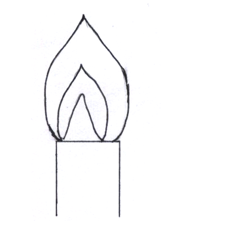a) Describe how this type of flame is produced.

b) Label on the diagram the least hot part of the flame.

c) Name the gas produced by a burning candle that is a non –pollutant

3 marks

2.

The element X can be represented as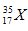a) How many neutrons are contained in X?

b) Use the data in the table below to calculate the relative atomic mass of X from the masses and percentage abundance.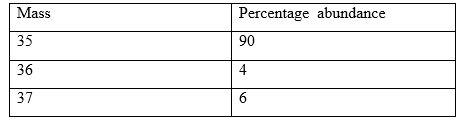3 marks

3.

a) Write a chemical equation for the reaction that occur when Carbon (iv) oxide gas is bubbled in calcium hydroxide solution.

b) Give a reason why Potassium hydroxide solution is not used to identify carbon (iv) oxide in the laboratory.

3 marks

4.

Below are standard electrode potentials for two half cells.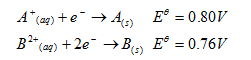i) Write the cell represented when the two half cells are combined to give an electrochemical cell. ________________________________________________________________________ _______________ ________________________________________________________________________ _______________

ii) Write the cell equation for the reaction that occur when the two half cells are combined.

iii) Calculate the e.m.f of the electrochemical cell formed from A and B.

3 marks

5.

Study the diagram below and answer the questions that follow.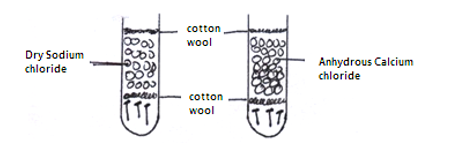a) State and explain the observations made after two weeks.

b) Give one reason for Silver plating an Iron spoon.

3 marks

6.

In a neutralization reaction, Sodium carbonate solution was dissolved in water and the solution made to 250cm3. 25cm3 of this solution neutralized 20cm3 of 0.25Mnitric acid. Calculate the mass of Sodium carbonate that was dissolved in water.

3 marks

7.

A chromatogram of enzymes A and E and three simple sugars are shown below.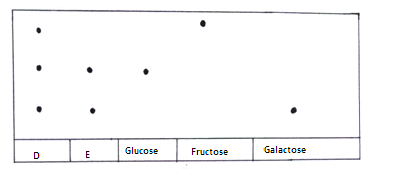Which two simple sugars are present in D and E.

3 marks

8.

When a piece of burning magnesium is lowered into a gas jar of sulphur (iv) oxide it continues to burn for sometime. a) State and explain the observations made

b) Write a chemical equation for the reaction which occurred.

4 marks

9.

a) A hydrocarbon consists of 92.3% carbon. It’s molecular mass is 26. Calculate it’s molecular formula.

b) Draw the structure of the hydrocarbon.

3 marks

10.

Study the flow chart below and answer the questions that follow: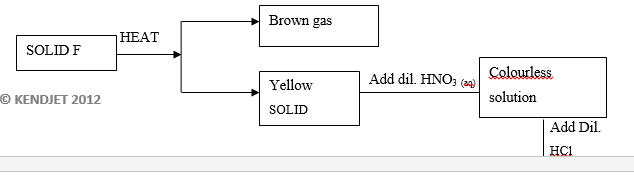ntify the cation and anion present in solid F.

I. Cation ______________________________________________________________________________ ________________ ______________________________________________________________________________ ________________

II. Anion ______________________________________________________________________________

b) Write ionic equation for the formation of the white precipitate.

3 marks

11.

Hydrogen sulphide gas is slightly soluble in water. The reaction is given by equation belowState and explain the effect of addition of Potassium hydroxide pellets on the concentration of hydrogen sulphide.

3 marks

12.

a) Calculate the enthalpy of formation of Carbon (ii) oxide from the data below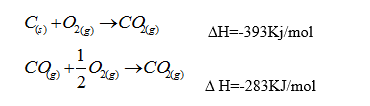b) State one disadvantage of using Carbon (II) oxide as a fuel.

3 marks

13.

a) Explain why melting point of chlorine gas is greater than that of Argon. ______________________________________________________________________________ ________________ ______________________________________________________________________________ ________________

b) Using dot( ) and cross to represent electrons draw a diagram to show bonding in carbon (iv) oxide.

c) In terms of structure explain why Graphite is used as a lubricant.

3 marks

14.

The diagram below show what’s produced when Nitrogen (iv) oxide gas is passed over heated Zinc granules.i) Identify gas H.

ii) Write an equation for the reaction that produces gas H.

iii) Using oxidation numbers, explain why the production of gas H is a reduction process.

3 marks

15.

. Given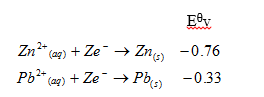Draw an electrochemical cell indicating the direction of electron flow.

3 marks

16.

Given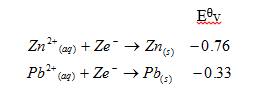Draw an electrochemical cell indicating the direction of electron flow.

4 marks

17.

An organic compound J reacts with Sodium hydroxide to form another with the formula: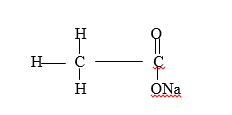a) Name the compound J.

b) Write an equation to show how reaction occurs.

c) Give one use of compound J.

3 marks

18.

At 400C, 25cm3 of aqueous Potassium nitrate has a concentration of 0.22 moles. Calculate the solubility of the Potassium nitrate. (K=39,N=14,O=16)

3 marks

19.

Starting with distilled water and solid Lead (II) nitrate; describe how a sample of Lead (II) carbonate can be prepared.

3 marks

20.

On the grid provided, sketch curves of volume of Hydrogen gas produced against time when Magnesium ribbon reacts with 1M,1.5M and 2M hydrochloric acid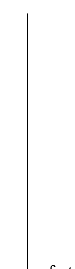3 marks

21.

In the manufacture of Sodium by electrolysis, why is Calcium chloride added to the molten electrolyte?

1 marks

22.

When a piece of Magnesium ribbon is placed a) in 1M Hydrochloric acid, bubbles of a colourless gas re produced more vigorously than in 1M Ethanoic acid. Explain

3 marks

23.

When a piece of Magnesium ribbon is placed

a) in 1M Hydrochloric acid, bubbles of a colourless gas re produced more vigorously than in 1M Ethanoic acid. Explain

b) Write ionic equation for the reaction between magnesium and 1M hydrochloric acid.

3 marks

24.

Study the table below.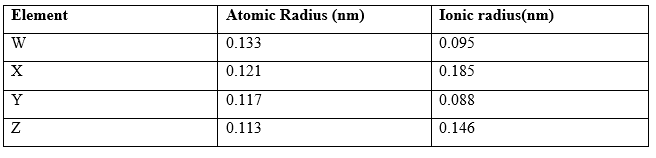a) Identify the most reactive non-metal. Explain.

b) Name the type of bond in a compound formed between W and Z.

3 marks

25.

Study the diagram showing extraction of Sulphur from underground deposit.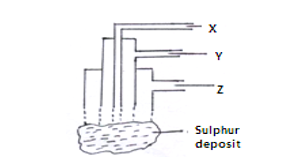a) i) State the role of substance X.

ii) Name substances Y and Z.

b) Give one use of sulphur.

3 marks

26.

When 0.8g of Ammonium nitrate was dissolved in 100cm3 of water, the temperature changed from 240C to 220C.Calculate the molar enthalpy of dissolution. N=14,H=1,O=16; specific heat capacity of water is 4.2Jg-1K-1; density of water is 1gcm-3

3 marks

27.

When a few drops of aqueous ammonia is added to solution A, a white precipitate is formed. The white precipitate dissolves when excess ammonia solution is added.

a) Identify the cation present in solution A.

b) Write ionic equation for the production of the white precipitate. ______________________________________________________________________________ ________________ ______________________________________________________________________________ ________________

c) Give the formula of the complex ion formed when excess ammonia solution is added to solution A.

3 marks

28.

a) What is observed when a few drops of phenolphthalein indicator is added to a solution whose pH value is 3.0?

b) Write an equation for the reaction between: i) Lead (ii) oxide and dilute Nitric acid

ii) Lead (ii) oxide and Sodium hydroxide solution.

3 marks

29.

a) Study the equation below and use it to determine the type of water hardness being removed.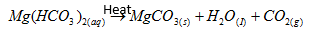b) Stated one disadvantage of water hardness.

3 marks

Back Top